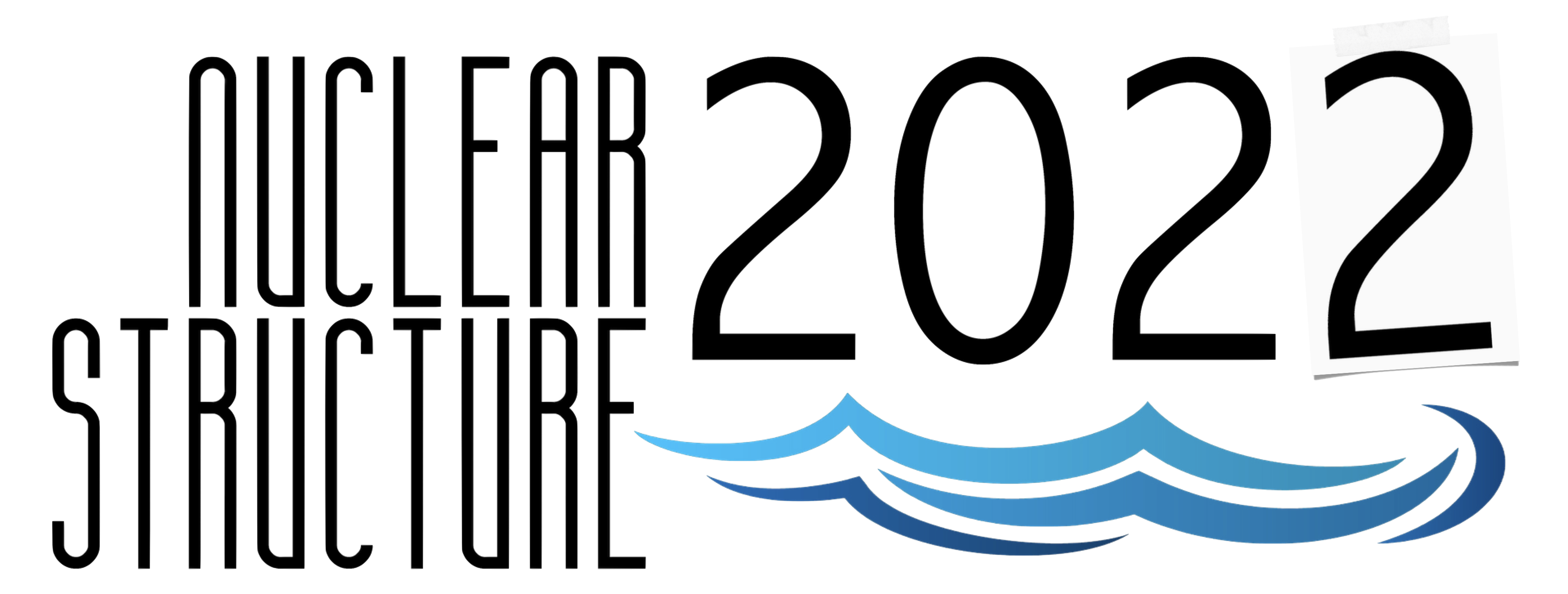#Nuclear Structure 2022

Jun 13 – 17, 2022
Berkeley, CA
US/Pacific timezone

## The band structure at high spin in $^{61}$Ni

Not scheduled
1m
Berkeley, CA

#### Berkeley, CA

Lawrence Berkeley National Laboratory
Oral Poster Presentations

### Speaker

Soumik Bhattacharya (Florida State University)

### Description

Ni nuclei, having the magic proton number Z=28, are observed to have spherical shapes near ground state excitation throughout the N=28 to N=40 shell-closure. The experimentally observed low and moderate energy states can be well reproduced by the shell-model calculations involving $\nu$p$_{3/2}$, $\nu$f$_{5/2}$, $\nu$p$_{1/2}$ and $\nu$g$_{9/2}$ orbitals[1,2]. Though the N=40 sub-shell closure is observed to exist in $^{68}$Ni, the energy gap between the p$_{1/2}$ and g$_{9/2}$ orbitals are estimated to be of the order of 2$~$MeV only. This small sub-shell gap allows the cross-shell excitations in $^{68,67}$Ni[1,4] and the moderate excited states can be reproduced by shell-model calculations promoting a neutron to the $\nu$0g$_{9/2}$ across the N=40 shell gap. The involvement of shape driving $\nu$0g$_{9/2}$ orbital induce collectivity in lighter mid-shell Ni isotopes and rotational bands have been reported in $^{56-59}$Ni[5-8]. Furthermore, with more excitation energy, magnetic rotational bands and super-deformed bands were also observed in $^{60}$Ni and $^{63}$Ni respectively. The magnetic rotational band in $^{60}$Ni has been explained as multi-quasiparticle excitation involving a proton hole in high-j f$_{7/2}$ and neutron particle in another high-j g$_{9/2}$. The neighbouring nuclei $^{61}$Ni with an extra valance neutron are therefore expected to exhibits the similar collective excitations. Recently, the excited states in $^{61}$Ni has been studied experimentally with $^{7}$Li beam but the well deformed states at high spin were not observed.

The present work has been able to produce the $^{61}$Ni in much higher excitation energy and spin with $^{50}$Ti($^{14}$C,3n)$^{61}$Ni reaction using enriched thin $^{50}$Ti foil at 43$~$MeV beam energy from the 9$~$MV Tandem accelerator at the Fox laboratory, Florida State University (FSU). The de-excited $\gamma$-rays were detected using the FSU array of 6 HPGe clover detectors with BGO shields and several single crystal HPGe detectors placed at 3 different angles; 90$^{\deg}$, 45$^{\deg}$ and 135$^{\deg}$. The $\gamma-\gamma$ coincidence analysis are performed to establish the level scheme. Further, the DCO ratio and polarization measurements are utilized to assign the spin-parity of the excited levels. The present work has considerably extended the level scheme up to 13$~$MeV and $31/2\hbar$ spin with the observation of 25 new levels and 46 new transitions decaying from them in $^{61}$Ni with assignment of definite spin and parity for the most of the newly established excited states. We have observed three band structure on the intrinsic single particle levels. Two of these bands are of dipole nature whereas the other one is formed with quadrupole (E2) transitions. The lower states of the $^{61}$Ni can be well explained as the coupling of one valance neutron, occupying the available p$_{3/2}$, f$_{5/2}$, p$_{1/2}$ and g$_{9/2}$, with the low lying 0$^{+}$, 2$^{+}$ and 4$^{+}$ states of even-even $^{60}$Ni core. The dipole bands with the sequences of M1+E2 transitions, observed in $^{61}$Ni, may be interpreted as magnetic rotational band as it already seen as in adjacent $^{60}$Ni. The regular E2 structure is interpreted as rotational band with small deformation.

Reference:
 R. Broda et. al., Phys. Rev. C 86, 064312 (2012).
 J. P. Schiffer et. al., Phys. Rev. C 87, 034306 (2013).
 M. Bernas et. al., Phys. Lett. B 113, 279 (1982).
 S. Zhu et. al., Phys. Rev. C 85, 034336 (2012).
 E. K. Johansson et. al., Phys. Rev. C 77, 064316 (2008).
 D. Rudolph et. al., J. Phys. G: Nucl. Part. Phys. 37, 075105 (2010).
 E. K. Johansson et. al., Phys. Rev. C 80, 014321 (2009).
 C.-H. Yu et. al., Phys. Rev. C 65, 061302(R) (2002).
 D. A. Torres et. al., Phys. Rev. C 78, 054318 (2008).
 M. Albers et. al., Phys. Rev. C 80, 054314 (2013).
 S. Samanta et. al., Phys. Rev. C 99, 014315 (2019).

### Primary authors

Soumik Bhattacharya (Florida State University) Dr Vandana Tripathi (Florida State University, Tallahassee, Florida 32306, USA) Mr S. Ajayi (Florida State University, Tallahassee, Florida 32306, USA) Rebeka Sultana Lubna (TRIUMF) Dr Elizabeth Rubino (National Superconducting Cyclotron Laboratory) Caleb Benetti (Florida State University) Prof. Samuel Tabor (Florida State University) Yutaka Utsuno (Advanced Science Research Center, Japan Atomic Energy Agency) Mr Noritaka Shimizu (Center for Nuclear Study, the University of Tokyo, Hongo, Bunkyo-ku, Tokyo 113-0033, Japan ) J.M. Allmond (Oak Ridge National Laboratory)

### Presentation materials

There are no materials yet.